### A sort of swirly curvy map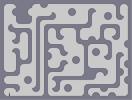Hover over the thumbnail for a full-size version.

Author wilde author:wilde tileset unrated 2010-08-28 2010-08-28 5 more votes required for a rating. \$A sort of swirly curvy map#wilde#tileset#9000000000000000619619600000;<00000000001001008;<006187<0;800071001801111<0111=0:11111=00:=011111019000006190000000111110180000071000;<0001961=0:1111111=000:=000900000000000000000007<0000;11111111111111111=0<0019000000000000000007=0010;11111111111111111007101900619:=06190000600:=01800010000010;87<000000111<018000710111=000000196=0:1<0;1=019000000001000006=0:90010;<00;<00100000000000010:=00:=0018000000;<00010000000001111<000:=000100000000019061800000071000700007100011111111110;110;111=00;190000611101960:900000:10;<00011=010;00000000710:=000190010:00000;111100000018001<000;<019061807<00:=001=000:=01000:111=00000010;80000187<0000000000710:111<0:11=000;1111111=00906=00000000:900:=00000800000000;<000;<00;<007|5^57.4817036410186,566# Um I can't do a good gameplay with it. So feel free to steal the tileset and make a fun level with it, as long as you credit me for the tiles ^_^

## Other maps by this author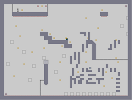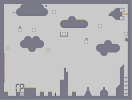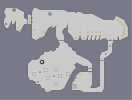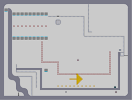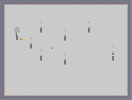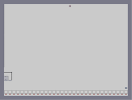AGD Challenge 2 The Attack Of The Giant Ninja Cragg's Cavern part I Speedrun Challenge AGD Challenge 3 Speedrun Challenge 2